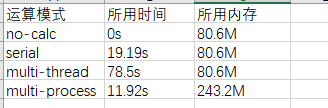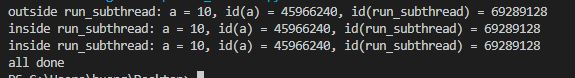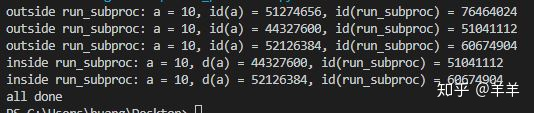• 不进行计算
• 串行运算
• 多线程运算
• 多进程运算

得到的结果如下：https://www.cnblogs.com/yssjun/p/11302500.html

#!/usr/bin/python
from multiprocessing import Process
import time
import sys

def test_func(n):
for _ in range(n):
for i in a:
j = i + 1
print j

a =  * 10000000 # 10M
ncore = 2
per_run = 20
total_run = ncore * per_run

if __name__ == “__main__”:
start_time = time.time()
if len(sys.argv) < 2:
print “Usage: python %s <no-calc | serial | multi-thread | multi-process>” % sys.argv
sys.exit(1)
cores = None
if sys.argv == “no-calc”:
time.sleep(1)
elif sys.argv == “serial”:
test_func(total_run)
cores = [Thread(target = test_func, args=(per_run, )) for _ in range(ncore)]
elif sys.argv == “multi-process”:
cores = [Process(target = test_func, args=(per_run, )) for _ in range(ncore)]
else:
print “Usage: python %s <no-calc | serial | multi-thread | multi-process>” % sys.argv
sys.exit(3)
if cores:
for cr in cores:
cr.start()
for cr in cores:
cr.join()
end_time = time.time()
print end_time – start_time

import time

a = 10

if __name__ == “__main__”:
t.start()
t.join()
print “all done”from multiprocessing import Process
import time

# each subprocess has its own copy of data.
def run_subproc(proc_id):
time.sleep(proc_id + 1)
print(“inside run_subproc: a = %d, d(a) = %d, id(run_subproc) = %d” % (a, id(a), id(run_subproc)))

a = 10
print(“outside run_subproc: a = %d, id(a) = %d, id(run_subproc) = %d” % (a, id(a), id(run_subproc)))

if __name__ == “__main__”:
proc = [Process(target=run_subproc, args=(idx, )) for idx in range(2)]
for p in proc:
p.start()
for p in proc:
p.join()
print “all done”